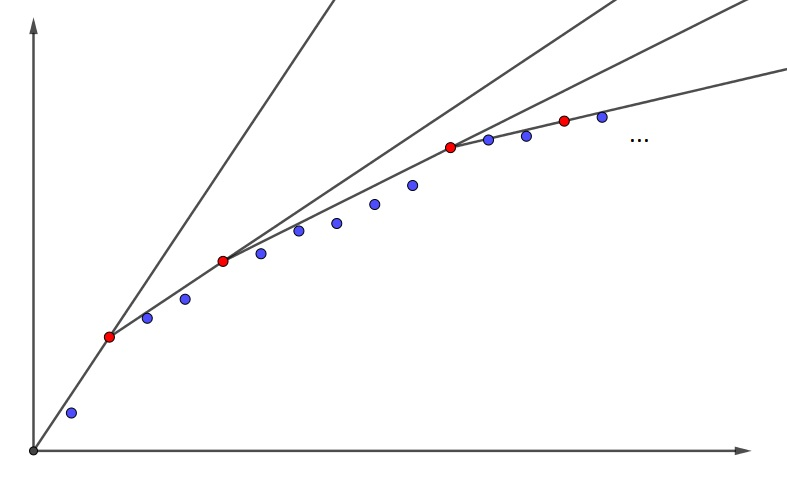Mathematical and Physical Journal
for High Schools
Issued by the MATFUND Foundation
 Already signed up? New to KöMaL?

#Problem A. 712. (December 2017)

A. 712. We say that a strictly increasing positive real sequence $\displaystyle a_1,a_2,\dots$ is an elf sequence if for any $\displaystyle c>0$ we can find an $\displaystyle N$ such that $\displaystyle a_n<cn$ for $\displaystyle n=N,N+1,\dots\,.$ Furthermore, we say that $\displaystyle a_n$ is a hat if $\displaystyle a_{n-i}+a_{n+i}<2a_n$ for $\displaystyle 1\le i\le n-1$. Is it true that every elf sequence has infinitely many hats?

(5 pont)

Deadline expired on January 10, 2018.

Proof without words:### Statistics:

 9 students sent a solution. 5 points: Daróczi Sándor, Gáspár Attila, Imolay András, Janzer Orsolya Lili, Matolcsi Dávid, Schrettner Jakab, Schweitzer Ádám, Szabó Kristóf. 4 points: Bukva Balázs.

Problems in Mathematics of KöMaL, December 2017